# Grade - math word problems

#### Number of problems found: 5458

• PerimetersA rectangle has a perimeter of 16p centimeters, it had a width of 2p centimeters. Each side of an equilateral triangle is 1/2 the length of the rectangle. Find the total perimeter of the rectangle and the triangle if p=8.
• Discount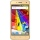The new phone was discounted by 2800kč. After this discount, 5 phones cost 5530 CZK more than 3 phones before the discount. How much did the phone cost before the discount?On the menu are 12 kinds of meal. How many ways can we choose four different meals into the daily menu?
• Gold, silver, bronzeHow many ways can we divide gold, silver, bronze medails if there are 6 people competing?
• Two numbers 7The sum of two consecutive even numbers is 30. Find the numbers.There are 15 boys and 12 girls at the graduation party. Determine how many four couples can be selected.
• Aircraft angines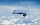The two engines of the aircraft are enough to supply the fuel for five hours of operation. However, one of the engines has a malfunction and thus consumes one-third more fuel. How long can the plane be in the air before it runs out of fuel? After an hour
• Surface area of a cubeWhat is the surface area of a cube that has an edge of 3.5?
• Roots and coefficient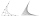In the equation 2x ^ 2 + bx-9 = 0 is one root x1 = -3/2. Determine the second root and the coefficient b.
• A cloth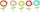There are flower cloth 495 meters, white cloth 330 meters. What is the percentage of white cloth? How much is white cloth less than flower cloth?
• 45th birthdayThis year Mrs. Clever celebrated her 45th birthday. Her three children are now 7.11 and 15 years old. In how many years will Mrs. Clever's age be equal to the sum of her children's years?
• Sheep and cowsThere are only sheep and cows on the farm. Sheep is eight more than cows. The number of cows is half the number of sheep. How many animals live on the farm?
• Tickets1260 tickets sold. On the first day, 80% was sold on the second day was sold. How many tickets were sold first and how much the next day?
• Video gameNicole is playing a video game where each round lasts 7/12 of an hour. She has scheduled 3 3/4 hours to play the game. How many rounds can Nicole play?
• Two machinesThe first machine can produce parts in 24 hours, the second in 16 hours. The first started working in the morning at 6:00 pm. Second at 10:00 o'clock. At what hour were all parts made?
• 1 page1 page is torn from the book. The sum of the page numbers of all the remaining pages is 15,000. What numbers did the pages have on the page that was torn from the book?
• BoatsThree-quarters of boats are white, 1/7 are blue and 9 are red. How many boats do we have?
• Sand castle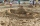Tim and Tom built a sand castle and embellished it with a flag. Half the pole with the flag plunged into the castle. The highest point of the pole was 80 cm above the ground, its lowest point 20 cm above the ground. How high was the sand castle?
• Workers 9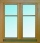If two workers fix two windows in an hour how many windows can 18 workers fix in the same two windows?
• Cuboid and ratioFind the dimensions of a cuboid having a volume of 810 cm3 if the lengths of its edges coming from the same vertex are in ratio 2: 3: 5

Do you have an interesting mathematical word problem that you can't solve it? Submit a math problem, and we can try to solve it.

We will send a solution to your e-mail address. Solved examples are also published here. Please enter the e-mail correctly and check whether you don't have a full mailbox.

Please do not submit problems from current active competitions such as Mathematical Olympiad, correspondence seminars etc...